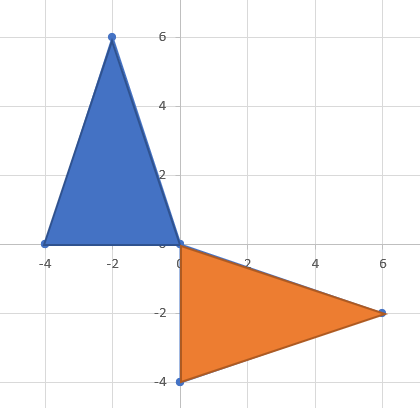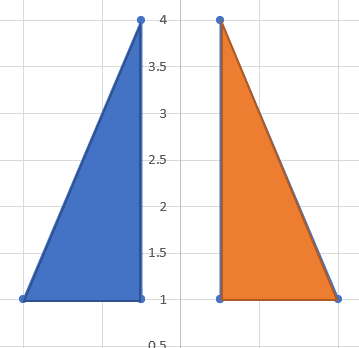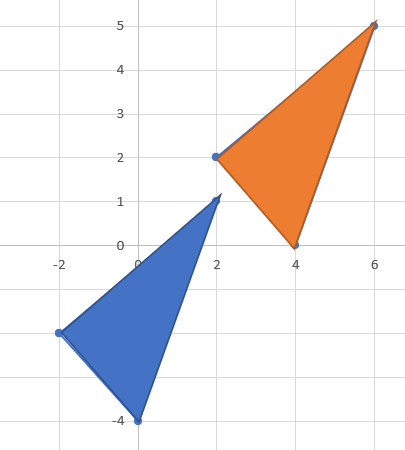# Congruent Triangles

Congruent Triangles are sets of triangles that have the exact same shapes and sizes. This means that they share side lengths and angles. The main difference between congruent triangles is how they are oriented, either due to turns, flips, and/or slides. This causes the triangles to look differently from each other, even though they're actually the same!#### Turns (Rotation)#### Flips (Relfection)#### Slides (Translation)

There are several different ways to determine whether a set of triangles are congruent to each other without knowing everything about either one. We need at least 3 values to determine their congruency (or lack thereof).

## SSS (Side, Side, Side)

If 3 side lengths of 2 different triangles are exactly equal to one another, they can be considered congruent.

Example
$$AB = DE$$
$$BC = EF$$
$$AC = DF$$

Therefore, we can determine that $$△\text{ABC} \;=\; △\text{DEF}$$.

## SAS (Side, Angle, Side)

If 2 side lengths and the included angle of 2 different triangles are exactly equal to each other, they can be considered congruent.

Example
$$∠H = ∠K$$
$$IH = JK$$
$$GH = JK$$

Therefore, we can determine that $$△\text{GHI} \;=\; △\text{JKL}$$.

## ASA (Angle, Side, Angle)

If 2 angles and the included side length of 2 different triangles are exactly equal to each other, they can be considered congruent.

Example
$$∠R = ∠U$$
$$∠S = ∠V$$
$$RS = UV$$

Therefore, we can determine that $$△\text{RST} \;=\; △\text{UVW}$$.

## AAS (Angle, Angle, Side)

If 2 angles and the non-included side length of 2 different triangles are exactly equal to each other, they can be considered congruent.

Example
$$∠A = ∠G$$
$$∠E = ∠K$$
$$AC = GI$$

Therefore, we can determine that $$△\text{ACE}\; = \;△\text{GIK}$$.

## HL (Hypoteneuse, Leg)

This method only applies to right-angled triangles. If the hypoteneuse and one of the legs in 2 different triangles are exactly equal to each other, they can be considered congruent.

Example
$$LN = OQ$$
$$NM = QP$$

Therefore, we can determine that $$△\text{MNO} \;=\; △\text{PQR}$$.

* When investigating if a set of triangles are congruent, you CANNOT use the following methods:

• AAA (Angle, Angle, Angle)
• SSA (Side, Side, Angle)
• ASS (Angle, Side, Side)

With AAA, 2 triangles can have the exact same angles but be different sizes. These triangles would then be considered Similar Triangles. You wouldn't be able to use any of these methods since there isn't enough information to determine if a set of triangles are congruent to each other.